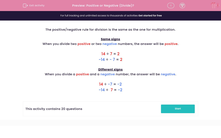# Positive or Negative (Divide)?

In this worksheet, students decide whether the answer to a division is positive or negative.This content is premium and exclusive to EdPlace subscribers.Key stage:  KS 2

Curriculum topic:   Maths and Numerical Reasoning

Curriculum subtopic:   Mixed Problems

Difficulty level:#### Worksheet Overview

The positive/negative rule for division is the same as the one for multiplication.

Same signs

When you divide two positive or two negative numbers, the answer will be positive.

14 ÷ 7 = 2

-14 ÷ - 7 = 2

Different signs

When you divide a positive and a negative number, the answer will be negative.

14 ÷ -7 = -2

-14 ÷  7 = -2

### What is EdPlace?

We're your National Curriculum aligned online education content provider helping each child succeed in English, maths and science from year 1 to GCSE. With an EdPlace account you’ll be able to track and measure progress, helping each child achieve their best. We build confidence and attainment by personalising each child’s learning at a level that suits them.

Get started